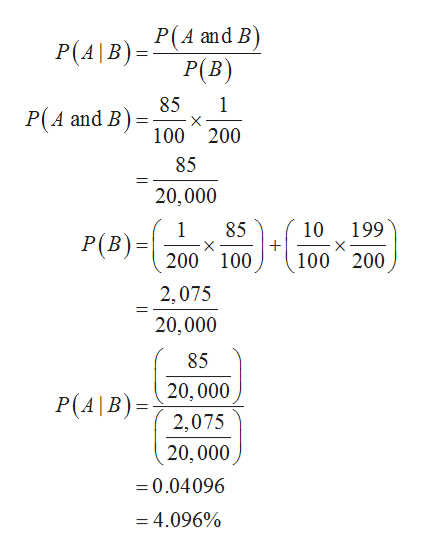# A certain virus infects one in every 200 people. A test used to detect the virus in a person is positive 85% of the time if the person has the virus and 10% of the time if the person does not have the virus. (This 10% result is called a false positive.) Let A be the event "the person is infected" and B be the event "the person tests positive".a) Find the probability that a person has the virus given that they have tested positive, i.e. find P(A|B). Round your answer to a percent rounded nearest fourth decimal place and do not include a percent sign.P(A|B)= %b) Find the probability that a person does not have the virus given that they test negative, i.e. find P(A'|B'). Round your answer as a percent rounded to the nearest fourth decimal place and do not include a percent sign.P(A'|B') = %

Question
76 views

A certain virus infects one in every 200 people. A test used to detect the virus in a person is positive 85% of the time if the person has the virus and 10% of the time if the person does not have the virus. (This 10% result is called a false positive.) Let A be the event "the person is infected" and B be the event "the person tests positive".

a) Find the probability that a person has the virus given that they have tested positive, i.e. find P(A|B). Round your answer to a percent rounded nearest fourth decimal place and do not include a percent sign.
P(A|B)= %

b) Find the probability that a person does not have the virus given that they test negative, i.e. find P(A'|B'). Round your answer as a percent rounded to the nearest fourth decimal place and do not include a percent sign.
P(A'|B') = %

check_circle

star
star
star
star
star
1 Rating
Step 1

It is given that one in every 200 people were infected by the virus.

Let the event A represent “the person is infected” and the event B represent “the person tests positive”.

That is, the probability of the person is infected is, P(A) = 1/200

The probability of the person is not infected is, P(A') = 199/200

For having virus:

P(True Positive) = 85%

P(True negative) = 15%

For not having virus:

P(False Positive) = 10%

P(False Negative) = 90%.

Step 2

a) Probability that a person has the virus given that they test positive:

The probability that a person has the virus given that they test positiv...help_outlineImage TranscriptioncloseP(A|B) PA and B P(B) 85 P(A and B) 1 X 100 200 85 20,000 199 1 85 P(B)=200 100 10 X X 100 200 2,075 20,000 85 20,000 P(A\B)= 2,075 20,000 =0.04096 =4.096% fullscreen

### Want to see the full answer?

See Solution

#### Want to see this answer and more?

Solutions are written by subject experts who are available 24/7. Questions are typically answered within 1 hour.*

See Solution
*Response times may vary by subject and question.
Tagged in

### Other